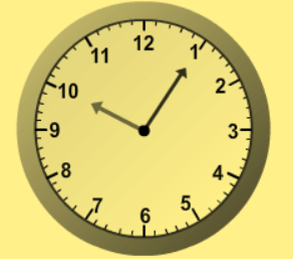1
visibility

What time does the clock below show?• A

11:05

• B

9:05

• C

1:05

• D

10:05

The correct answer is 10:05

Solution:

Consider the clock below:• The hour hand is between 10 and 11, so it is 10 hours.
• The minute hand is at 1. So, if we begin at 12 and count up by fives until we reach 1. It is 5 minutes.

So, the time shown by the clock is 10:05